Home MonkeyNotes Printable Notes Digital Library Study Guides Study Smart Parents Tips College Planning Test Prep Fun Zone Help / FAQ How to Cite New Title Request

2.4  Left Hand And Right Hand Limits ( One-sided Limits )

While defining the limit of a function f as x tends to 'a', we consider values of f (x) when x is very closed to 'a'. The values of x may be greater or lesser than a. If we restrict x to values less than a, we have seen that it is x + a- or x ® (a - 0). The limit of f with this restriction on x, is called the 'Left Hand Limit'. Similarly if x takes only values greater than a, as seen in x ® a+ or x ® a + 0. The limit of f is then called the 'right hand limit'.

Definition :A function 'f' is said to tend to limit l as x tends to a   from the left, if for each e > 0 (however small), there exists d > 0

such that | f(x) - l | > e when a - d < x < a

In symbols, we then write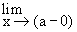f (x) = l   or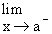f (x) = l    or   f (a - 0) = l or   f (a-) = l

Definition :A function 'f' is said to tend to a limit l as x tends to a from right, if for each e > 0 (however small), there exists d> 0

such that | f (x) - l | < e when a < x < a + d

In symbols, we then write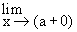f(x) = l    or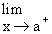f (x) = l   or    f (a + 0) = l or   f (a+) = l

These limits are called as 'one-sided' limits. It may be noted that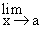f(x) exists if and only if both these limits, left hand and right hand, exist and are equal.

If the function 'f'does not approach a real number 'x® a' as x approaches a, then the limit does not exist. Then we write it asf (x) DNE (Does not exist).Your browser does not support the IFRAME tag.

Definition : After the intuitive approach, we now turn our attention to the rigorous definition.

Let a function 'f' be defined at every point of some neighborhood of a, except possibly at a. Then a real number l is called the limit of f at a, if for any e > 0 (however small), we can find a d > 0, such that

| f (x) - l | < e, whenever 0 < | x - a | < d

We write this asf (x) = l

Illustration : Let f(x) =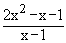for x ¹ 1

Then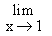f(x)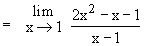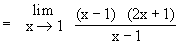=(2x + 1) = 3

Now when x ¹ 1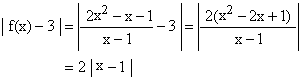Suppose we are given  a  positive number 0.002,  then  when  x  ¹ 1

| f(x) - 3 | = 2 | x - 1 | < 0.02. If | x-1 | < 0.01,

similarly for any given positive real number e,

| f(x) - 3 | < e, if | x - 1 |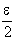< for x ¹ 1   Let= d

Then | f(x) - 3 |< e, if 0 < | x - 1 | < d

i.e.f (x) = 3

Index

2.1 Modulus
2.2 Inequalities
2.3 Limits Of Functions
2.4 Left Hand And Right Hand Limits
2.5 Theorems On The Algebra Of Limits
2.6 Evaluating Limits
2.7 Limits Of Trigonometric Functions
2.8 The Exponential Limits

Chapter 3